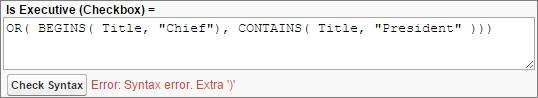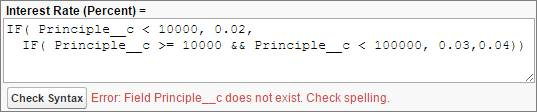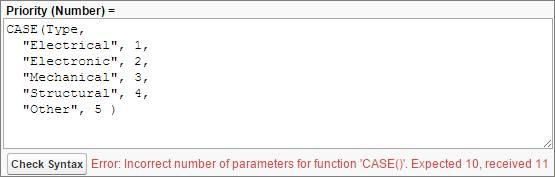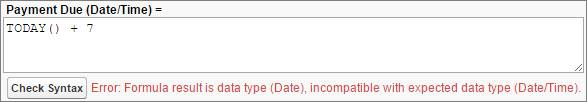Time Estimate

# Troubleshoot Formula Errors

## Learning Objectives

After completing this unit, you’ll be able to:
• Understand the causes of common formula errors.
• Troubleshoot advanced formula fields.

## Syntax Errors

Even advanced formula gurus run into problems with their formulas occasionally. The most common formula errors are syntax errors. To check the syntax of your formula at any time, click Check Syntax.

## Missing or Extra Parentheses

It’s easy to accidentally type an extra parenthesis in your formula or leave one out. This error is especially common when nesting logical statements such as IF(), AND(), and OR().

This formula, for example, is missing a close parenthesis at the end.You also get this error if you have too many parentheses or a comma out of place within a function. This is the same formula, but with an extra parenthesis.## Field Does Not Exist

When it comes to syntax errors, the Advanced Formula Editor is your friend. It’s almost impossible to misspell the name of a field or a function when you’re using the Insert Operator or Insert Field button.Here we meant to reference the field Principal__c, not Principle__c. You also get this error if you forget to put quotation marks around a Text string.

## Incorrect Number of Parameters

If you use the incorrect number of parameters for a certain function, you run into syntax errors. Be extra careful with functions that take a variable number of parameters, like CASE().This formula was missing the last argument, the fall-through case, in its CASE() statement. The Formula Editor assumed that we meant to check only four cases, not five. So it looked for 10 arguments in total, when we really meant to give it 12.

## Unknown Function

You also encounter errors when you misspell a function name or try to use a function that doesn’t exist.This formula attempts to reference the function MINIMUM(), which doesn’t exist. We meant to use MIN(), which takes a list of numbers and returns the minimum.

## Working with Different Data Types

When creating a formula, think of what type of data you want it to return before you write your formula. If your formula returns a data type other than the one you selected, you can’t save it.

It’s easy to mix up data types when converting a value between types or using similar data types, like Date and Date/Time or Number and Currency. This formula, for example, is written to return a Date value when the selected formula return type is Date/Time.To correct this error, you can change the return type of the formula field to Date. Or you can replace TODAY() with NOW() to produce a Date/Time value instead of a Date.

## Compile Size and Formula Length Errors

Formula fields are powerful, but restricted in size. Formulas are limited to 3,900 characters or 4,000 bytes, including spaces, return characters, and comments, and can’t exceed 5,000 bytes when compiled. It’s important to understand the differences between these size restrictions and how to work around or within the constraints.

## Length Limit

Formulas are limited to 3,900 characters. You can shorten long formulas in several ways. Replacing AND() with &&, for example, saves a few characters with each use, as does replacing nested IF() statements with a CASE() statement. Shorter field names and comments also make a small but significant difference in the length of your formula.

If your formula field is significantly longer than 3,900 characters, use a helper formula field.

## Compile Size Limit

A formula that is less than 3,900 characters can still exceed the 5,000-byte compile size limit. When a formula is over the compile size limit, creating helper fields and shortening field names or comments doesn’t make a difference. When you reference a helper field, its compile size is added to the compile size of the formula that references it. One way to reduce a formula’s compile size is by minimizing references to other formula fields.

Some methods for reducing formula length, such as replacing nested IF() statements with a CASE() statement, also reduce a formula’s compile size.

Consider two fields:
• Date1__c is a Date field.
• Date2__c is a formula field that creates a date from Date1__c.
`DATE( YEAR( Date1__c ), MONTH( Date1__c ), DAY( Date1__c ) )`

The following formula returns the date of the last day of the month for a given date (assume February always has 28 days):

```DATE(
YEAR( SomeDate__c ),
MONTH( SomeDate__c ),
IF(
OR(
MONTH( SomeDate__c ) = 4,
MONTH( SomeDate__c ) = 6,
MONTH( SomeDate__c ) = 9,
MONTH( SomeDate__c ) = 11
),
30,
IF(
MONTH( SomeDate__c ) = 2,
28,
31
)
)
)```

The formula first checks for months with 30 days, then February, and the remaining months are 31 days. It requires a nested IF() function, which isn’t very readable and compiles to 1069 characters for Date1__c and a whopping 7,271 characters for Date2__c! Why? Because the formula references the date seven times. Compare that to this revised version of the formula:

```DATE(
YEAR( SomeDate__c ),
MONTH( SomeDate__c ),
CASE(
MONTH( SomeDate__c ),
2, 28,
4, 30,
6, 30,
9, 30,
11, 30,
31
)
)```

Not only is this easier to read, the formula compiles to only 645 characters for Date1__c and 3,309 characters for Date2__c, and it now references the date three times instead of seven.

This example came from the Salesforce Answers community . A picklist stores the name of an agent responsible for an opportunity. The formula calculates a commission, based on Base Commission value and a multiplier. But because Base_Commission__c is referenced in each condition of the CASE() statement, the formula exceeds the compile size.

```CASE( Agent__c,
"John", Base_Commission__c * 2,
"Jane", Base_Commission__c * 6,
/* Repeat for many other agents */
Base_Commission__c
)```

To fix this, move Base_Commission__c outside the CASE() function. The formula can be rewritten as:

```Base_Commission__c * CASE( Agent__c,
"John", 2,
"Jane", 6,
/* Repeat for many other agents */
1
)```

Even if Base Commission is only a Currency field and not a formula itself, referencing it once instead of multiple times greatly reduces the formula compile size.

## ResourcesKeep learning for
free!
Sign up for an account to continue.
What’s in it for you?
• Get personalized recommendations for your career goals
• Practice your skills with hands-on challenges and quizzes
• Track and share your progress with employers
• Connect to mentorship and career opportunities